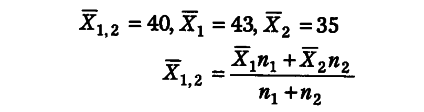# A book seller has 150 books of Economics and Accountancy

A book seller has 150 books of Economics and Accountancy. The average price of these books is ?40 per book. Average price of books on Economics is Rs.43 and that of Accountancy is Rs.35. Find the number of books on Economics with the seller.

Let n_{ 1 } = Economics; n_{ 2 } = Accountancy
Now, n_{ 1 } +n_{ 2 } = 150
n_{ 2 } = 150 - n_{ 1 }40 = 43\$n_{ 1 }\$ + 35(150-n_{ 1 })/150
40 = 43\$n_{ 1 }\$ + 5250 - 35\$n_{ 1 }\$/150
40 = 8\$n_{ 1 }\$+5250/150
6000 = 8\$n_{ 1 }\$+5250
8\$n_{ 1 }\$ = 750
====> n_{ 1 } = 750/8 = 93.75 = 94 (approx)
Thus, books on Economics with the seller are 94.